# Mathematics Support

### Rational Number Operations

Building Understanding of Rational Number Operations

Integrating Rational Number Operations in Expressions & Equations

## Building Understanding of Rational Number Operations

### Students will be able to...

*Instructional videos in the hyperlinks above are meant to support C2.0 content, but may use vocabulary or strategies not emphasized by MCPS.

The Common Core State Standards require a balance of three fundamental components that result in rigorous mathematics acquisition: deep conceptual understanding, procedural skill, and mathematical applications and modeling.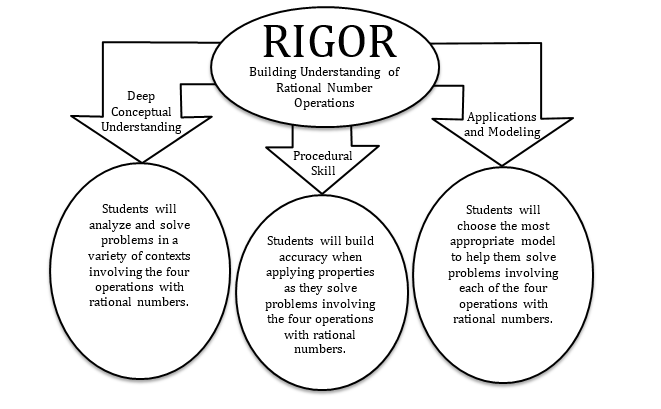### In school, your child will…

• Apply and extend previous understandings of addition and subtraction to add and subtract rational numbers; represent addition and subtraction on a horizontal or vertical number line diagram.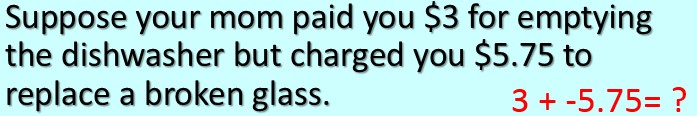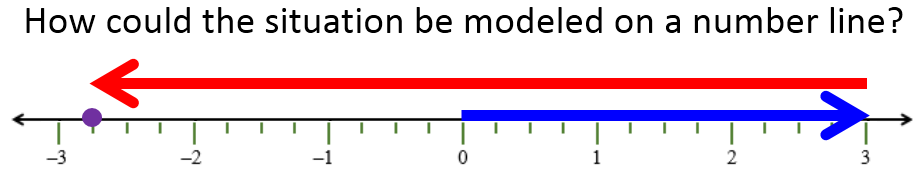• Apply and extend previous understandings of multiplication and division of fractions to multiply and divide rational numbers.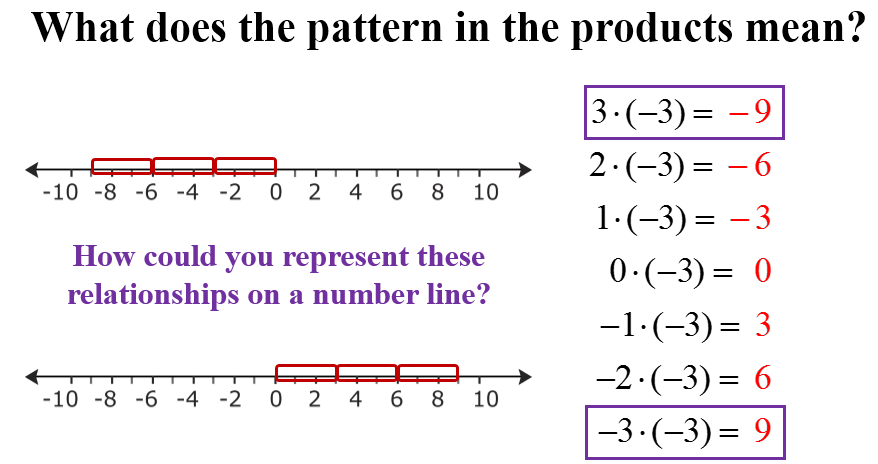• Solve real-world and mathematical problems involving the four operations with rational numbers.
• Jackie left home on Saturday and hiked 3.25 miles north, kayaked 5.5 miles south, and then biked 1.75 miles north. How many miles from home is she now?

• Apply properties of operations to calculate with numbers in any form; convert between forms as appropriate; and assess the reasonableness of answers using mental computation and estimation strategies.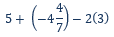### At home, your child can…

• Determine the amount of money in a checking account given a series of withdrawals and deposits.
• Determine the final price of a group of item after applying a given discount and sales
• Follow a stock for a week and model the gains/losses on a number line. Determine the net value of the stock from the first day until the end of the week.

*Additional Practice links support C2.0 content, but may use vocabulary or strategies not emphasized by MCPS.

## Integrating Rational Number Operations in Expressions & Equations

### Students will be able to...

• Apply properties of operations as strategies to add, subtract, factor, and expand linear expressions with rational coefficients.

• Understand that rewriting an expression in different forms in a problem context can shed light on the problem and how the quantities in it are related.
• Solve multi-step real-life and mathematical problems posed with positive and negative rational numbers in any form, using tools strategically. Apply properties of operations to calculate with numbers in any form; convert between forms as appropriate; and assess the reasonableness of answers using mental computation and estimation strategies.
• Use variables to represent quantities in a real-world or mathematical problem, and construct simple equations and inequalities to solve problems by reasoning about the quantities.

*Instructional videos in the hyperlinks above are meant to support C2.0 content, but may use vocabulary or strategies not emphasized by MCPS.

The Common Core State Standards require a balance of three fundamental components that result in rigorous mathematics acquisition: deep conceptual understanding, procedural skill, and mathematical applications and modeling.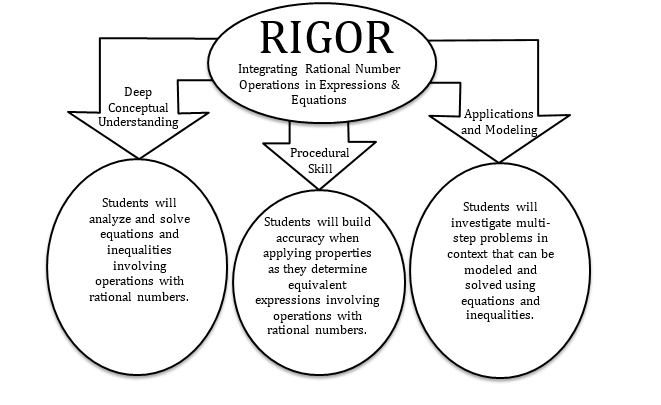### In school, your child will…

• Apply properties of operations as strategies to add, subtract, factor, and expand linear expressions with rational coefficients.

Write three algebraic expressions that are equivalent to the model shown below: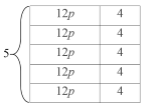• Understand that rewriting an expression in different forms in a problem context can shed light on the problem and how the quantities in it are related.

A group of friends are going to see the same movie and purchase the same snack. If a ticket costs \$8.25 each and snacks cost \$2.25 each, what expressions model the scenario?

• Solve multi-step real-life and mathematical problems posed with positive and negative rational numbers in any form, using tools strategically. Apply properties of operations to calculate with numbers in any form; convert between forms as appropriate; and assess the reasonableness of answers using mental computation and estimation strategies.

If you want to place a towel bar 9 3/4 inches long in the center of a door that is 27 1/2 inches wide, where will you need to place the bar?

• Use variables to represent quantities in a real-world or mathematical problem, and construct simple equations and inequalities to solve problems by reasoning about the quantities.

The perimeter of a rectangle is 54 cm. Its length is 6 cm. What is its width?

### At home, your child can...

• Determine an equation or inequality that can describe a real world problem.

Find the number of tickets that can be purchased for an event, including the ticket surcharge. We have \$100 to go to the ballgame. If tickets cost \$26.50 each and we want to have \$20 to buy snacks, how many tickets can we buy?

• After a shopping trip, write and solve equation that can be used to determine the cost of the items.

For example, last week we bought three spiral notebooks and a pencil that costs \$0.75. If we gave the cashier \$10.00 and received \$4.75 in change, how much did each notebook cost?